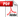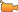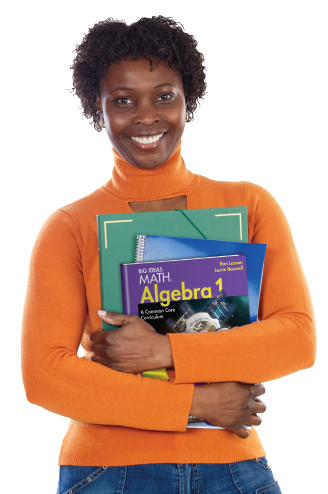# Standards for Mathematical Practice

Big Ideas Math is a research-based program, systematically developed using the Common Core State Standards for Mathematical Practice as the underlying structure. Throughout the program the Standards for Mathematical Practice are seamlessly connected to the Common Core State Content Standards resulting in a program that maximizes both teacher effectiveness and student understanding.

## Mathematically Proficient Students

1  Make sense of problems and persevere in solving them.Poster for Mathematical Practice #1

Each lesson begins with an Essential Question. Students look for entry points using guides such as Think About the Question and In Your Own Words. Clear stepped-out examples encourage students to plan a solution pathway rather than jumping into a solution attempt. Guided questions and scaffolding support students' perseverance.

2  Reason abstractly and quantitatively.Watch the Big Ideas Math video for Mathematical Practice #2Poster for Mathematical Practice #2

Throughout the series students are expected to model, deduce, and conjecture. Essential Questions, Error Analysis, and Reasoning exercises provide opportunities for students to make assumptions, examine results and explain their reasoning. What Is Your Answer, In Your Own Words, You Be The Teacher, and Which One Doesn't Belong encourage debate and sense making.

3  Construct viable arguments and critique the reasoning of others.Watch the Big Ideas Math video for Mathematical Practice #3Poster for Mathematical Practice #3

In each lesson, students work with the mathematics of everyday life. Students are asked to write stories involving math, such as using percents to help them improve their grades. Students use graphs, tables, charts, number lines, diagrams, flowcharts, and formulas to organize, make sense of and identify realistic solutions to real-life situations. Visual representations, such as integer tiles and fraction models are used to help students make sense of numeric operations.

4  Model with mathematics.Watch the Big Ideas Math video for Mathematical Practice #4Poster for Mathematical Practice #4

Students learn to represent problems by consistently using a verbal model, paying close attention to units and properties. This helps students represent problems symbolically and manipulate the representative symbols. They are taught to contextualize by thinking about the referents and symbols involved.

5  Use appropriate tools strategically.Watch the Big Ideas Math video for Mathematical Practice #5Poster for Mathematical Practice #5

Opportunities for students to select and use appropriate tools such as graphing calculators, protractors, measuring devices, websites, and other external resources are provided for students throughout the series.6  Attend to precision.Watch the Big Ideas Math video for Mathematical Practice #6Poster for Mathematical Practice #6

Through the balanced approach to instruction students have the opportunity to communicate mathematically daily. Students work through activities, examples, and exercises to understand and use the language of mathematics paying careful attention to the importance of units, labeling, and quantities.

7  Look for and make use of structure.Watch the Big Ideas Math video for Mathematical Practice #7Poster for Mathematical Practice #7

Real and relevant word problems are posed to encourage students to "see" these problems as being composed of several objects. Students see that some mathematical representations share common mathematical structures and learn to look for these relationships discerning inherent patterns and structures.

8  Look for and express regularity in repeated reasoning.Watch the Big Ideas Math video for Mathematical Practice #8Poster for Mathematical Practice #8

The program helps students see that mathematics is well structured and predictable. Students are encouraged to work through a problem, not through the numbers. They consider factors such as what the problem is asking, are the intermediate steps reasonable, and is the solution realistic.